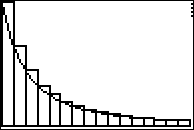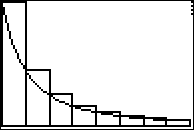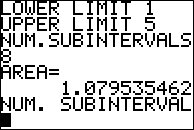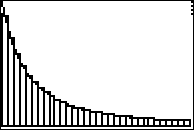••• ##### Device
• TI-83 Plus Family
• TI-84 Plus
• TI-84 Plus Silver Edition
•TI-84 Plus C Silver Edition
•TI-84 Plus CE
• ##### Software

TI Connect™
TI Connect™ CE

• ##### Report an Issue

Calculus: Approximating Integrals with Riemann Sums
by Texas Instruments#### Overview

In this activity, students calculate Riemann sums. They demonstrate when the Riemann sums will over-approximate a definite integral. They also observe the convergence of Riemann sums as the number of subintervals get larger.

#### Key Steps

•Students will calculate and analyze Reimann sums.

•Students will use a calculator program to automate the process of calculating the sum.

•Students will observe the convergence of left-hand and right-hand Riemann sums by using graphing
handhelds to automate the production of Riemann sums with regular partitions having a large number of subintervals.